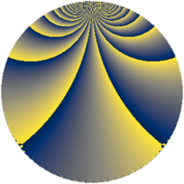# Properties

 Label 456.3.sLevel $456$ Weight $3$ Character orbit 456.s Rep. character $\chi_{456}(107,\cdot)$ Character field $\Q(\zeta_{6})$ Dimension $312$ Sturm bound $240$

# Related objects

## Defining parameters

 Level: $$N$$ $$=$$ $$456 = 2^{3} \cdot 3 \cdot 19$$ Weight: $$k$$ $$=$$ $$3$$ Character orbit: $$[\chi]$$ $$=$$ 456.s (of order $$6$$ and degree $$2$$) Character conductor: $$\operatorname{cond}(\chi)$$ $$=$$ $$456$$ Character field: $$\Q(\zeta_{6})$$ Sturm bound: $$240$$

## Dimensions

The following table gives the dimensions of various subspaces of $$M_{3}(456, [\chi])$$.

Total New Old
Modular forms 328 328 0
Cusp forms 312 312 0
Eisenstein series 16 16 0

## Trace form

 $$312q - 6q^{3} - 4q^{4} + 7q^{6} - 2q^{9} + O(q^{10})$$ $$312q - 6q^{3} - 4q^{4} + 7q^{6} - 2q^{9} + 24q^{10} + 12q^{16} - 40q^{19} + 18q^{22} - 53q^{24} - 704q^{25} + 30q^{28} + 136q^{30} + 48q^{33} + 36q^{34} - 35q^{36} + 144q^{40} - 16q^{42} - 4q^{43} + 63q^{48} - 1896q^{49} - 6q^{51} + 114q^{52} - 16q^{54} + 18q^{57} + 40q^{58} - 174q^{60} + 704q^{64} + 31q^{66} - 12q^{67} + 720q^{70} - 237q^{72} - 84q^{73} + 620q^{76} + 402q^{78} + 54q^{81} - 54q^{82} + 330q^{90} + 576q^{91} - 442q^{96} - 12q^{97} + 32q^{99} + O(q^{100})$$

## Decomposition of $$S_{3}^{\mathrm{new}}(456, [\chi])$$ into newform subspaces

The newforms in this space have not yet been added to the LMFDB.